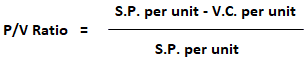Contribution Margin Ratio: Definition

The contribution margin ratio is the total contribution margin divided by total sales. It is also known as the profit-volume (P/V) ratio.

Contribution Margin Ratio: Explanation

The contribution margin is the amount of revenue in excess of variable costs. One way to express it is on a per-unit basis, such as standard price (SP) per unit less variable cost per unit.

The contribution margin may also be expressed as a percentage of sales. When the contribution margin is expressed as a percentage of sales, it is called the contribution margin ratio or profit-volume ratio (P/V ratio).

Formula to Calculate Contribution Margin Ratio

The contribution margin (or P/V) ratio is calculated as follows:Example

Company X manufactures and sells only one product. The per-unit costs are:

SP per unit = \$30
VC per unit = \$18
Contribution margin per unit = \$12

The company reports fixed costs of \$40,000.

Required: Calculate the contribution margin ratio (or P/V ratio).

P/V ratio = 30 – 18/30 = 40%

A contribution margin ratio of 40% means that 40% of the revenue earned by Company X is available for the recovery of fixed costs and to contribute to profit. The remaining 60% is consumed by variable costs.

The break-even point (BEP) is calculated using the contribution margin ratio. The formula is:

BEP = Fixed costs / P/V ratio

= 40,000 / 40%
= \$100,000

Conceptually, the contribution margin ratio reveals essential information about a manager’s ability to control costs. This is especially true in relation to sales revenue.

A firm’s ability to make profits is also revealed by the P/V ratio. With a high contribution margin ratio, a firm makes greater profits when sales increase and more losses when sales decrease compared to a firm with a low ratio.